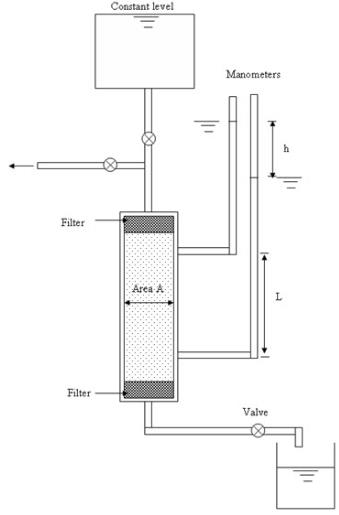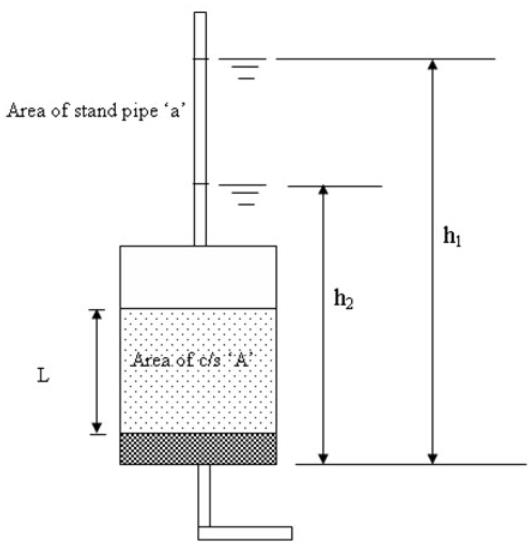Courses

# Agricultural Engineering Notes | EduRev

## Agricultural Engineering : Agricultural Engineering Notes | EduRev

The document Agricultural Engineering Notes | EduRev is a part of the Agricultural Engineering Course Soil Mechanics Notes- Agricultural Engineering.
All you need of Agricultural Engineering at this link: Agricultural Engineering

Introduction
The coefficient of permeability can be determined by laboratory as well as field tests and by empirical approach. In the laboratory, coefficient of permeability is determined by constant head and falling head test. In the field, permeability is determined by unconfined and confined flow pumping tests.

Constant head test is suitable for coarse grained soil as sufficient discharge is required to determine the coefficient of permeability. Figure 18.1 shows the schematic diagram of constant head permeameter. The water is allowed to flow through the soil sample from a reservoir such a way that a constant water level is maintained in the reservoir by overflow. The quantity of water (Q) flowing through the soil for a particular time (t) or discharge is measured. The coefficient of permeability is determined as:

$k={{QL} \over {Aht}}cm/s$            (18.1)

where

k = coefficient of permeability (cm/s)

Q = discharge collected in time t (cm3/s)

A = c/s area of the sample (cm2)

h = head drop (distance) in manometers levels (cm)

L = distance between manometer tapping point (cm)Falling head test is suitable for fine sands, silts. Figure 18.2 shows the schematic diagram of falling head permeameter. The water is allowed to flow through the soil sample and the height difference of the water level in the stand pipe for a particular time interval is measured. The coefficient of permeability is determined as:

$k=2.3{{aL} \over {At}}{\log _{10}}{{{h_1}} \over {{h_2}}}$                (18.2)

where

k = coefficient of permeability (cm/s)

A = c/s area of the sample (cm2)

a= area of the stand pipe (cm2)

h = distance in manometers levels (cm)

h1 (cm) and h2 (cm) = height of the water level in stand pipe in time difference t (s)

Unconfined Pumping Tests

Figure 18.3 shows the unconfined pumping test procedure. Here aquifer is underlain by an impermeable layer and pumping well is extended up to the bottom of the permeable layer as shown in Figure 18.3. Two observation wells are inserted in the aquifer. The permeability is determined as:

$k=2.3{q \over \pi }{{{{\log }_{10}}({r_2}/{r_1})} \over {(h_2^2 - h_1^{2)}}}$                       (18.3)

where q is discharge, k is coefficient of permeability, h1 and h2 can be determined by measuring the drawdown at the observation wells (as shown in the Figure 18.3).  rand rare the distance of the observation well from the pumping well.Offer running on EduRev: Apply code STAYHOME200 to get INR 200 off on our premium plan EduRev Infinity!

64 docs

,

,

,

,

,

,

,

,

,

,

,

,

,

,

,

,

,

,

,

,

,

;